# Sequences and Series Class 11

In Sequences and Series class 11 chapter 9 deals with the study of sequences which follow a specific pattern called progression. In this chapter, the concepts such as arithmetic progression (A.P),  geometric mean, arithmetic mean, the relationship between A.M. and G.M., special series in forms of the sum to n terms of consecutive natural numbers, sum to n terms of squares and cubes of natural numbers will also be studied.

## Sequence and Series Class 11 Concepts

The topics and subtopics covered in chapter 9 – Sequences and Series class 11 concepts are:

• Introduction
• Sequences
• Series
• Arithmetic Progression (A.P.)
• Arithmetic mean
• Geometric Progression (G . P.)
• The general term of a G .P.
• Sum to n terms of a G .P.
• Geometric Mean (G .M.)
• Relationship Between A.M. and G.M.
• Sum to n Terms of Special Series

## Sequences and Series Class 11 Notes

The different numbers occurring in any particular sequence are known as its terms. The terms of a sequence are denoted by

a1, a2, a3,….,an

If a sequence has a finite number of terms then it’s known as a finite sequence. A sequence is termed as infinite if it is not having a definite number of terms. The nth term of an AP is given by

a + (n-1) d.

Between any two numbers ‘a’ and ‘b’, n numbers can be inserted such that the resulting sequence is an Arithmetic Progression. A1 , A2 , A3,……,An be n numbers between a and b such that a, A1 , A2 , A3,……,An, b is in A.P.

Here, a is the 1st term and b is (n+2)th term. Therefore,

b = a + d[(n + 2) – 1] = a + d (n + 1).

Hence, common difference (d) = (b-a)/(n+1)

Now, A1= a+d= a+((b-a)/(n+1)),

A2= a+2d = a+ ((2(b-a)/(n+1)),

An =a+nd= a+ ((n(b-a)/(n+1))}

The nth term of a geometric progression is given by a= arn-1

### Sequences and Series class 11 Examples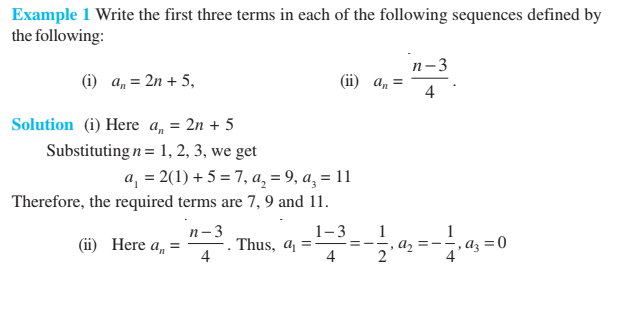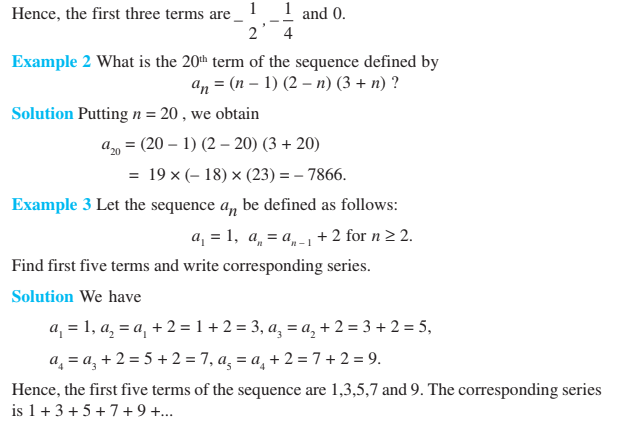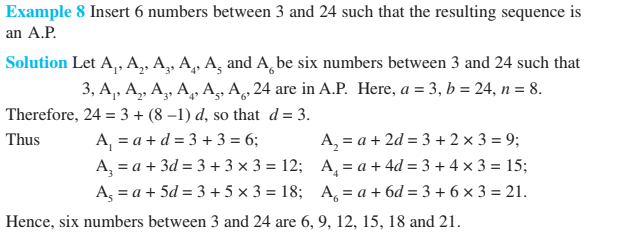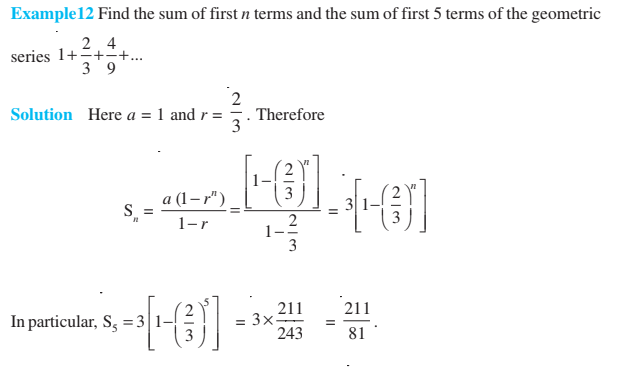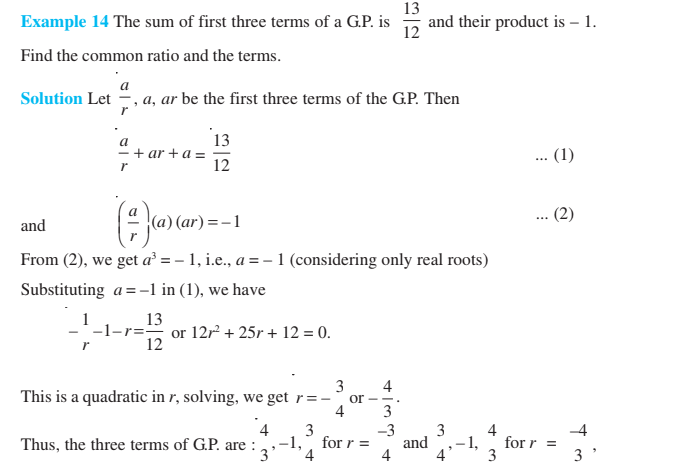Keep visiting BYJU’S – The Learning App and also download the app to get more class-wise concepts with examples and personalized videos.

 For More Class 11 Concepts: Conic Sections Class 11 Complex Numbers Class 11 Limits and Derivatives Class 11 Linear Inequalities Class 11